# WA Inverse Undecimated Wavelet Transform VI

LabVIEW 2014 Advanced Signal Processing Toolkit Help

Edition Date: June 2014

Part Number: 372656C-01

»View Product InfoDownload Help (Windows Only)

Owning Palette: Discrete Wavelet VIs

Requires: Advanced Signal Processing Toolkit

Computes the multi-level inverse undecimated wavelet transform (UWT) and returns the reconstructed signal from the approximation coefficients and the detail coefficients. Wire data to the UWT coef input to determine the polymorphic instance to use or manually select the instance.

Examples

Use the pull-down menu to select an instance of this VI.

 Select an instance WA Inverse 1D Undecimated Wavelet Transform (Waveform)WA Inverse 1D Undecimated Wavelet Transform (Array)WA Inverse 2D Undecimated Wavelet Transform

## WA Inverse 1D Undecimated Wavelet Transform (Waveform)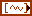UWT coef specifies the approximation coefficients and the detail coefficients for the multi-level inverse undecimated wavelet transform (UWT). You must organize the coefficients in an array of waveforms starting with the approximation coefficients at the largest level followed by the detail coefficients at all levels in descending order.wavelet specifies the wavelet type of the discrete wavelet analysis. The default is db02. wavelet must be the same type as the wavelet type you selected in the WA Undecimated Wavelet Transform VI to compute UWT coef. If you used the analysis filters input of the WA Undecimated Wavelet Transform VI to specify wavelet, ignore this input and use the synthesis filters input of this VI to specify the synthesis filters of the wavelet.error in describes error conditions that occur before this node runs. This input provides standard error in functionality.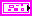synthesis filters specifies the coefficients of the lowpass synthesis filters and the highpass synthesis filters for the wavelet you specify. If you specify a value for synthesis filters, this VI ignores the settings in the wavelet input. You can use the Wavelet Design Express VI to design the analysis filters and the corresponding synthesis filters.lowpass specifies the coefficients of the lowpass synthesis filter, which this VI uses to filter the interpolated approximation coefficients in the wavelet reconstruction.highpass specifies the coefficients of the highpass synthesis filter, which this VI uses to filter the interpolated detail coefficients in the wavelet reconstruction.signal returns the reconstructed signal.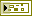error out contains error information. This output provides standard error out functionality.

## WA Inverse 1D Undecimated Wavelet Transform (Array)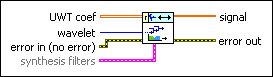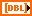UWT coef specifies the approximation coefficients and the detail coefficients for the multi-level inverse undecimated wavelet transform (UWT). You must organize the coefficients into a 2D real array starting with the approximation coefficients of the largest level at the first row followed by the detail coefficients of all levels in descending order.wavelet specifies the wavelet type of the discrete wavelet analysis. The default is db02. wavelet must be the same type as the wavelet type you selected in the WA Undecimated Wavelet Transform VI to compute UWT coef. If you used the analysis filters input of the WA Undecimated Wavelet Transform VI to specify wavelet, ignore this input and use the synthesis filters input of this VI to specify the synthesis filters of the wavelet.error in describes error conditions that occur before this node runs. This input provides standard error in functionality.synthesis filters specifies the coefficients of the lowpass synthesis filters and the highpass synthesis filters for the wavelet you specify. If you specify a value for synthesis filters, this VI ignores the settings in the wavelet input. You can use the Wavelet Design Express VI to design the analysis filters and the corresponding synthesis filters.lowpass specifies the coefficients of the lowpass synthesis filter, which this VI uses to filter the interpolated approximation coefficients in the wavelet reconstruction.highpass specifies the coefficients of the highpass synthesis filter, which this VI uses to filter the interpolated detail coefficients in the wavelet reconstruction.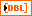signal returns the reconstructed signal.error out contains error information. This output provides standard error out functionality.

## WA Inverse 2D Undecimated Wavelet Transform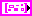UWT coef specifies the approximation coefficients and the detail coefficients for the multi-level inverse undecimated wavelet transform (UWT). Each element of the array contains the 2D UWT results of one level. The ith element stores the approximation coefficients and the detail coefficients at level i+1.low_low specifies the approximation coefficients from the lowpass analysis filtering on each row and each column. The low_low coefficients are a low-resolution approximation of the original 2D signal.low_high specifies the detail coefficients from the lowpass analysis filtering on each row and the highpass analysis filtering on each column. The high-frequency signal along the column direction influences the low_high coefficients.high_low specifies the detail coefficients from the highpass analysis filtering on each row and the lowpass analysis filtering on each column. The high-frequency signal along the row direction influences the high_low coefficients.high_high specifies the detail coefficients from the highpass analysis filtering on each row and each column. The high-frequency signal along the diagonal direction influences the high_high coefficients.wavelet specifies the wavelet type of the discrete wavelet analysis. The default is db02. wavelet must be the same type as the wavelet type you selected in the WA Undecimated Wavelet Transform VI to compute UWT coef. If you used the analysis filters input of the WA Undecimated Wavelet Transform VI to specify wavelet, ignore this input and use the synthesis filters input of this VI to specify the synthesis filters of the wavelet.error in describes error conditions that occur before this node runs. This input provides standard error in functionality.synthesis filters specifies the coefficients of the lowpass synthesis filters and the highpass synthesis filters for the wavelet you specify. If you specify a value for synthesis filters, this VI ignores the settings in the wavelet input. You can use the Wavelet Design Express VI to design the analysis filters and the corresponding synthesis filters.lowpass specifies the coefficients of the lowpass synthesis filter, which this VI uses to filter the interpolated approximation coefficients in the wavelet reconstruction.highpass specifies the coefficients of the highpass synthesis filter, which this VI uses to filter the interpolated detail coefficients in the wavelet reconstruction.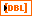signal returns the reconstructed signal.error out contains error information. This output provides standard error out functionality.

## Examples

Refer to the following VIs for examples of using the WA Inverse Undecimated Wavelet Transform VI:

• Undecimated Image Decomposition and Reconstruction (UWT) VI: labview\examples\Wavelet Analysis\WAGettingStarted
• Undecimated Signal Decomposition and Reconstruction (UWT) VI: labview\examples\Wavelet Analysis\WAGettingStarted

Not Helpful